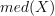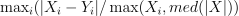# module `onnxrt.validate.validate_difference`#

## Short summary#

module `mlprodict.onnxrt.validate.validate_difference`

Validates runtime for many :scikit-learn: operators. The submodule relies on onnxconverter_common, sklearn-onnx.

source on GitHub

## Functions#

function

truncated documentation

`measure_relative_difference`

Measures the relative difference between predictions between two ways of computing them. The functions returns nan …

## Documentation#

Validates runtime for many :scikit-learn: operators. The submodule relies on onnxconverter_common, sklearn-onnx.

source on GitHub

mlprodict.onnxrt.validate.validate_difference.measure_relative_difference(skl_pred, ort_pred, batch=True, abs_diff=False)#

Measures the relative difference between predictions between two ways of computing them. The functions returns nan if shapes are different.

Parameters:
• skl_pred – prediction from scikit-learn or any other way

• ort_pred – prediction from an ONNX runtime or any other way

• batch – predictions are processed in a batch, skl_pred and ort_pred should be arrays or tuple or list of arrays

• abs_diff – return the absolute difference

Returns:

relative max difference or nan if it does not make any sense

Because approximations get bigger when the vector is high, the function computes an adjusted relative differences. Let’s assume X and Y are two vectors, let’s denotethe median of X. The function returns the following metric:.

The function takes the fourth highest difference, not the three first which may happen after a conversion into float32.

source on GitHub